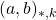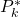# The unitary analogue of Pillai’s arithmetical functions. II

László Tóth
Notes on Number Theory and Discrete Mathematics, ISSN 1310-5132
Volume 2, 1996, Number 2, Pages 40—46

## Details

### Authors and affiliations

László Tóth
Department of Mathematics and Computer Science
“Babes-Bolyai” University
Str. M. Kogalniceanu 1
RO-3400 Cluj-Napoca, Romania

### Abstract

Let k be a positive integer and letdenote the greatest k-th power divisor of a which is a unitary divisor of b. We introduce the functionand obtain the arithmetical evaluation of it and an asymptotic formula for the summatory function of, which improves for k = 1 an earlier result of the author.

### References

1. J. Chidambaraswamy and R. Sitaramachandrarao, Asymptotic results for a class of arithmetical functions, Mh. Math. 99(1985), 19-27.
2. E. Cohen, Arithmetical functions associated with the unitary divisors of an integer, Math Z. 74(1960), 66-80.
3. B. Gordon and E. Rogers, Sums of the divisor function, Canadian J. Math. 16(1964), 151-158.
4. FI. G. Kopetzky, Bin asymptotischer Ausdruck fur eine zahlentheoretische Funktion, Mh. Math. 84(1977), 213-217.
5. K. Nageswara Rao, On the unitary analogues of certain totients, Mh. Math. 70(1966), 149-154.
6. S. S. Pillai, On an arithmetic function, J. Annamalai Univ. 2(1933), 243-248.
7. M. V. Subbarao and D. Suryanarayana, Sums of the divisor and unitary divisor functions, J. Reine Angew. Math. 302(1978), 1-15.
8. E. Teuffel, Aufgabe 599, Elem. Math. 25(1970), 65.
9. L. Toth, The unitary analogue of Pillai’s arithmetical function, Collect. Math. 40(1989), 19-30.

## Cite this paper

Tóth, L. (1996). The unitary analogue of Pillai’s arithmetical functions. II. Notes on Number Theory and Discrete Mathematics, 2(2), 40-46.